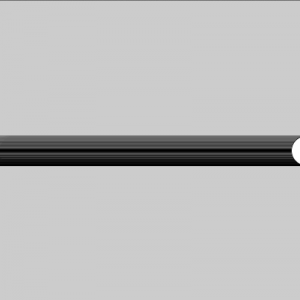# 002 – Processing Basics II

## I Making things move

So far we have been creating programs that run only once. In order to create animations or interactive software, we need to somehow make the program run continuously. Fortunately, Processing makes this pretty easy. It has built in functions called setup() and draw(). The setup() function runs once when the program starts and the draw() function keeps running on a loop until you quit the program. This is how you use them:

```void setup() {
}

void draw() {
}```

Now we have the functions in place, but they don’t actually do anything yet, since there is no code inside the functions. Anything that is between the curly braces { } after setup, is part of the setup function. Same thing applies for the draw function.

Also remember to write the word void in front of the setup and draw. We will come back to why that needs to be there later, but for now, you just need to remember that it should always be there. From now on, we should always start any program we write by first adding these two functions. The next step is to add some code inside the functions.

```void setup() {
// Set the size of the window
// This needs to be only done once
size(400, 400);
}

void draw() {
// draw an ellipse
ellipse(200, 200, 40, 40);
}```

It looks like nothing special is going on. There’s just an ellipse in the middle of the window. It looks like a still image, but actually the program is drawing the ellipse over and over 60 times a second. Let’s edit the code and make the ellipse move.

```// We will use a variable called 'x' to move the ellipse
// The initial value of the 'x' is 0
int x = 0;

void setup() {
// Set the size of the window
// This needs to be only done once
size(400, 400);
}

void draw() {
// draw an ellipse
// use the variable 'x' as the x-coordinate
ellipse(x, 200, 40, 40);

// Add 1 to the previous value of x
x = x + 1;
}```

When you run this sketch, you should see an ellipse moving on the x-axis leaving a weird smear on its path.Let’s take a closer look at the draw() function and figure out what is going on. This is the code without the comments:

```int x = 0;

void setup() {
size(400, 400);
}

void draw() {
ellipse(x, 200, 40, 40);
x = x + 1;
}```

The key to this is the ‘x’. In the beginning the value of ‘x’ is 0 and during the first fame of the program, the sketch draws the ellipse at (0, 200). After the ellipse is drawn, the program executes the following line:

`x = x + 1;`

Since ‘x’ at this point is 0 this would be the same as:

`x = 0 + 1;`

So on the next frame the ‘x’ would be 1 and the ellipse is drawn at (1, 200). Every frame 1 is added to the previous value of ‘x’ so the value just keeps growing. These are the x and y coordinates from the beginning of the program:

• Frame 1: (0, 200)
• Frame 2: (1, 200)
• Frame 3: (2, 200)
• Frame 4: (3, 200)
• ….

So it’s quite obvious why the ellipse is moving, but why does it leave this smear or feedback? Processing basically draws each frame on top of the previous one and since there is nothing that we are drawing on the background, the ellipses just sort of overlay on top of the previous ones. There is an easy fix for this if you don’t wish to have the feedback effect. We just need to clear the background on every frame.

```int x = 0;

void setup() {
size(400, 400);
}

void draw() {
background(255);
ellipse(x, 200, 40, 40);
x = x + 1;
}```

## III Bouncing Ball

Here is the example code for making an ellipse bounce around on the screen. We used the if statement to set conditions for the movement. This example does not have any gravity or friction so the movement of the ellipse looks very mechanical.

```// This code makes a ball bounce around.

float posX = 200; // X position of the circle
float posY = 60; // Y position of the circle

float speedX = 10; // X Speed
float speedY = 6; // Y speed

void setup(){
size(400,400);
}

void draw(){
background(50);

// Draw the ellipse
fill(255);
//noStroke();
ellipse(posX,posY,50,50);

// Each frame we add the speed to the X and Y position
posX = posX + speedX;
posY = posY + speedY;
//println(posX);

// If the circle hits the right side of the window...
if(posX >= 375){
// ...the X speed should reverse direction. (Multiply with -1.)
speedX = speedX * -1;
}
// If the circle hits the left side of the window...
if (posX <= 25){
// ...the X speed should once again flip. (Multiply with -1.)
speedX = speedX * -1;
}
// If the circle hits the bottom of the window...
if (posY >= 375){
// ...the Y speed should reverse. (Multiply with -1.)
speedY = speedY * -0.9;
}
// If the circle hits the top of the window...
if(posY <= 25){
// ...the Y speed should reverse. (Multiply with -1.)
speedY = speedY * -0.9;
}
}```

## III Random

You can create some simple, but interesting results by just using the random function.

```// Draw randomly placed lines

void setup() {
size(640,480);
background(255);
}

void draw() {
line(random(640), random(480), random(640), random(480));
}```